# Const and Read-Only in C#

## Const and Read-Only in C# with Examples

In this article, I am going to discuss the need and use of the keywords Const and Read-only in C# with examples. Please read our previous article where we discussed the Static and Non-Static Members in C# with examples. The const and read-only are very two useful keywords in C# and also a little confusing to understand. So, as part of this article, we are going to discuss the following pointers in detail.

1. Const Variable in C#.
2. Example using Const variable.
3. The read-Only variable in C#.
4. Example using the read-only variable.
5. Difference between Const, Readonly, Static and Non-Static Variable in C#.
##### According to MSDN

The Constants variables are the immutable values that are known at the time of program compilation and do not change their values for the lifetime of the program. The Read-only variables are also immutable values but these values are known at runtime and also do not change their values for the life of the program. With this definition keep in mind, let’s try to understand the const and readonly with some examples and let us try to understand the difference between them.

##### Constant Variables in C#:

In C#, if we declare a variable by using the const keyword, then it is a constant variable and the value of the constant variable can’t be modified once after its declaration. So, it is mandatory to initialize the constant variable at the time of its declaration only. Suppose, you want to declare a constant PI in your program, then you can declare the constant variable as follows:

const float PI = 3.14f;

If you are not initializing the const variable at the time of its declaration, then you will get a compiler error as shown in the below image.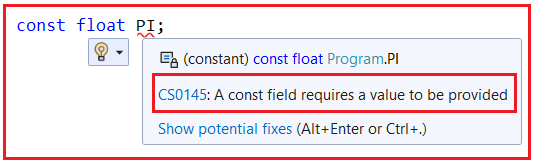As you can see it is saying a const field requires a value to be provided which means while declaring a constant it is mandatory to initialize the constant variable.

Note: The constant variables are going to be created one and only one time. This is because we cannot modify the constant values once after its declaration. If it allows creating multiple copies of the constant variable, then all those copies are going to store the same value which means it is a waste of memory. So, when we cannot modify a value, and if we are creating the same copy multiple times, then it is a waste of resources.

The behavior of constant variable is similar to the behavior of static variables i.e. initialized once and only one time in the life cycle of a class and does not require an instance of a class either for initialization or execution. For a better understanding, please have a look at the following example. The following code is self-explained, so please go through the comment lines.

##### Example to Understand Const Variable in C#

Let us understand the Const variable in C# with an example. Please have a look at the following example. As you can see in the below code, we declare a const variable i.e. const float PI = 3.14f; and within the Main method, we access the const variable by using the class name and can access it directly also. This is because const are static by default and as static it does not require an object instead it can be accessed by using the class name or directly within the same class. It is also possible to declare local variables as const i.e. we can declare const variable within a method also. In our example, within the Main method, we declare a const variable i.e. const int Number = 10; But once we declare the const variable, then we cannot change its value and if we try to change the value of the const variable in C#, then we will get a compile-time error.

```using System;
namespace ConstDemo
{
class Program
{
//we need to assign a value to the const variable
//at the time of const variable declaration else it will
//give compile time error
const float PI = 3.14f; //Constant Variable
static void Main(string[] args)
{
//Const variables are static in nature
//so we can access them by using class name
Console.WriteLine(Program.PI);
//We can also access them directly within the same class
Console.WriteLine(PI);

//We can also declare a constant variable within a function
const int Number = 10;
Console.WriteLine(Number);

//Once after declaration we cannot change the value
//of a constant variable. So, the below line gives an error
//Number = 20;

}
}
}
```
###### Output:##### Points to Remember while Working with Const Variable in C#:
1. The keyword const is used to create a “constant” variable. It means it will create a variable whose value is never going to be changed. In simple words, we can say that the variable whose value cannot be changed or modified once after its declaration is known as a constant variable.
2. Constants are static by default.
3. It is mandatory to initialize a constant variable at the time of its declaration.
4. The behavior of a constant variable is the same as the behavior of a static variable i.e. maintains only one copy in the life cycle of the class and initializes immediately once the execution of the class start (object not required)
5. The only difference between a static and constant variable is that the static variables can be modified whereas the constant variables in C# can’t be modified once it is declared.

As we already discussed constants variable should be assigned a value at the time of variable declaration and hence these values are known at compile time. So, whenever we declare a constant variable, the C# compiler substitutes its value directly into the Intermediate Language (MSIL). Let us understand this with an example. Please have a look at the following code. Here, we have created three variables. One is a constant, another one is a non-static variable and one is a static variable.

```using System;
namespace ConstDemo
{
class Program
{
public const float PI = 3.14f; //Constant Variable
int Age = 10; //Non-Static Variable
static string Message = "Good Morning"; //Static Variable
static void Main(string[] args)
{
}
}
}
```

Now, let us open the MSIL code of the above application using the ILDASM tool. Once you open the MSIL code of the above application, you will see the following. The following is the MSIL code of the Age variable which is a non-static variable. The value will be known as runtime and hence the value is not replaced at compile time in MSIL code.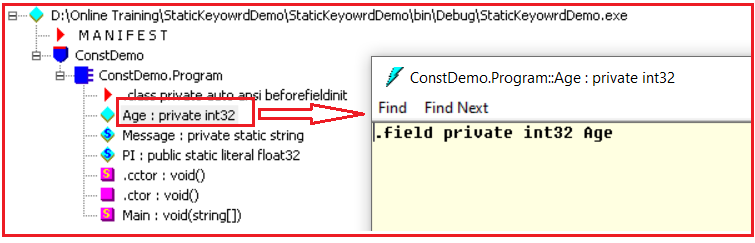The following is the MSIL code of the Name variable which is a static variable. The value will be known as runtime and hence the value is not replaced at compile time in MSIL code.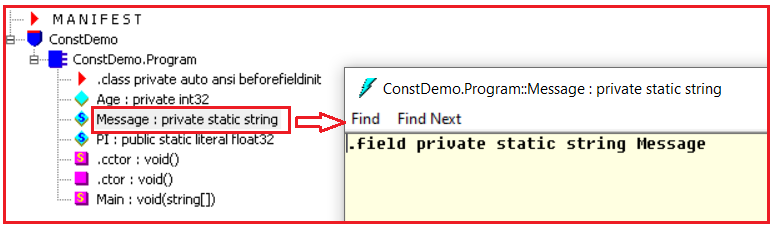The following is the MSIL code of the PI variable which is a constant variable. The value will be known at compile time and hence the value is replaced at compile time in the MSIL code.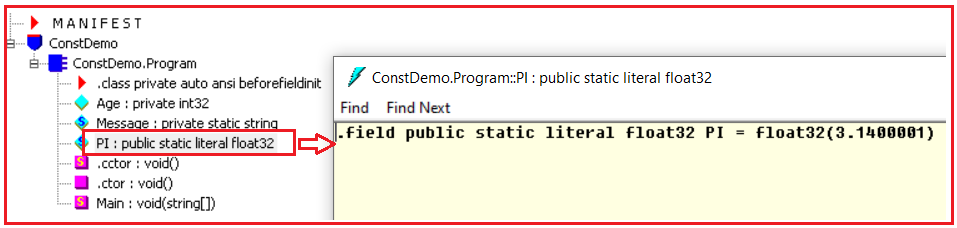You can see that the value 3.14 is replaced in the MSIL code. So, whenever we declare a constant variable, the C# compiler substitutes its value directly into the Intermediate Language (MSIL).

When we declare a variable by using the readonly keyword, then it is known as a read-only variable and these variables can’t be modified like constants but after initialization. That means it is not mandatory to initialize a read-only variable at the time of its declaration, they can also be initialized under the constructor. That means we can modify the read-only variable value only within a constructor.

The behavior of read-only variables will be similar to the behavior of non-static variables in C#, i.e. initialized only after creating the instance of the class and once for each instance of the class is created. So, we can consider it as a non-static variable, and to access readonly variables we need an instance.

##### Example to Understand Read-Only Variables in C#:

In the below example, the read-only variable x is not initialized with any value but when we print the value of the variable using the instance, the default value of int i.e. 0 will be displayed.

```using System;
namespace TypesOfVariables
{
internal class Program
{
static void Main(string[] args)
{
Program obj1 = new Program();
Console.WriteLine(\$"{obj1.x}");
}
}
}
```
##### Read-only Variable Initialization at the time of its Declaration in C#

Please have a look at the following example. As you can see in the below code, we declare a readonly variable i.e. readonly int number = 5; and within the Main method we access the readonly variable by using the object of the ReadOnlyExample. This is because readonly variables are non-static by default and as non-static it requires an object instead. Here, we have initialized the read-only variable with the value 5 at the time of its declaration and that value 5 will be displayed when we print the variable number.

```using System;
{
class Program
{
static void Main(string[] args)
{
Program obj = new Program();
Console.WriteLine(obj.number);
}
}
}
```
##### Read-only Variable Initialization through Constructor in C#

In the below example, we are initializing the readonly variable through the class constructors. You can directly initialize the readonly variables at the time of declaration or you can initialize through class constructors. Once initialized then you cannot change the value of readonly variables in C#.

```using System;
{
class Program
{

//You can also initialize through constructor
public Program()
{
number = 20;
}

static void Main(string[] args)
{
Program obj = new Program();
Console.WriteLine(obj.number);

//You cannot change the value of a readonly variable once it is initialized
//The following statement will give us compile time error
//obj.number = 20;

}
}
}
```
##### Readonly Variables are created once per Instance:

The read-only variables behavior is very much similar to the behavior of non-static variables in C#, i.e. initialized only after creating the instance of the class and once for each instance of the class is created. For a better understanding, please have a look at the below example. Here, the constructor takes one parameter and that parameter value we used to initialize the readonly variable. And inside the Main method, we are creating two instances of the class and we are initializing each instance of the read-only variable with a different value.

```using System;
{
class Program
{

public Program(int num)
{
number = num;
}

static void Main(string[] args)
{
Program obj1 = new Program(100);
Console.WriteLine(obj1.number);

//You cannot change the value of a readonly variable once it is initialized
//The following statement will give us compile time error
//obj1.number = 20;

Program obj2 = new Program(200);
Console.WriteLine(obj2.number);

}
}
}
```
###### Output: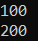As you can see in the above output, different values come out of the program’s output for the two different instances of the class. Hence it proves that read-only variables are also immutable values that are known at runtime and do not change their values for the life of the program.

##### Points to Remember while working with Read-Only Variable in C#:
1. The variable which is created by using the readonly keyword is known as a read-only variable in C#. The read-only variable’s value cannot be modified once after its initialization.
2. It is not mandatory or required to initialize the read-only variable at the time of its declaration like a constant. You can initialize the read-only variables under a constructor but the most important point is that once after initialization, you cannot modify the value of the readonly variable outside the constructor.
3. The behavior of a read-only variable is similar to the behavior of a non-static variable. That is, it maintains a separate copy for each object. The only difference between these two is that the value of the non-static variable can be modified from outside the constructor while the value of the read-only variable cannot be modified from outside the constructor body.
##### What is the difference between a constant and readonly variable in C#?

The difference between a constant and readonly variable in C# is that a constant is a fixed value for the whole class whereas readonly is a fixed value specific to an instance of a class and for each instance.

##### Const, Readonly, Static and Non-Static Variables Example in C#:

Let us understand Const, Readonly, Static and Non-Static variables in C# with one example. For a better understanding, please have a look at the following example.

```using System;
{
internal class Program
{
const float PI = 3.14f; //Constant Variable
static int x = 100; //Static Variable
//We are going to initialize variable y and z through constructor
int y; //Non-Static or Instance Variable

//Constructor
public Program(int a, int b)
{
//Initializing non-static variable
y = a;
z = b;
}

static void Main(string[] args)
{
//Accessing the static variable without instance
Console.WriteLine(\$"x value: {x}");
//Accessing the constant variable without instance
Console.WriteLine(\$"PI value: {PI}");

//Creating two instances
Program obj1 = new Program(300, 45);
Program obj2 = new Program(400, 55);
//Accessing Non-Static and Readonly variables using instance
Console.WriteLine(\$"obj1 y value: {obj1.y} and Readonly z value: {obj1.z}");
Console.WriteLine(\$"obj2 y value: {obj2.y} and Readonly z value: {obj2.z}");
}
}
}
```
###### Output: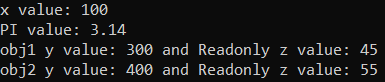For a better understanding of the above example, please have a look at the following diagram which shows the memory representation.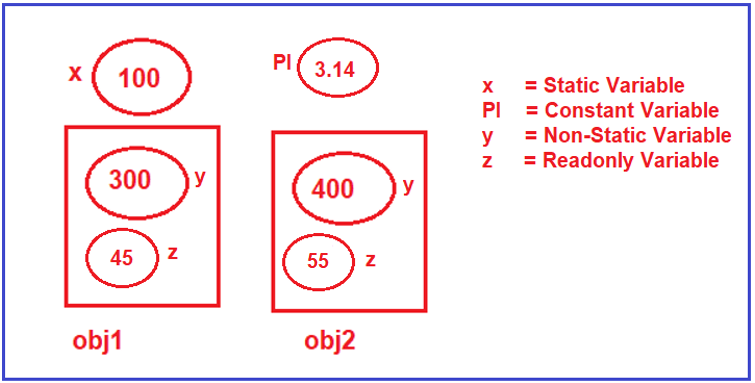### 9 thoughts on “Const and Read-Only in C#”

1.In last Example correct to
Console.WriteLine(obj1.flag + ” ” + obj2.flag);

1.false true

2.excellent

3.Good article

4.Your article with examples are very useful. One suggestion. At the end of article please give a summary with noted points about the concept discussed. That would be very helpful for us to recall the important points

1.We appreciate your suggestion, we will surely include the main points at the end of the article.

1.Still waiting for your action on the same. It will be great if you can provide a summary at the end of each article.

1.There are more than 5000 articles on the site. It will take more time to update all the articles with summary points. But we are working on this and will update the same in some time.

5.Great useful article .Nice job !!!